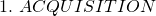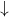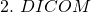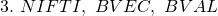A brief introduction to diffusion weighted MRI (DWMRI) processing

This post serves as a brief introduction to DWMRI processing.

dwmri.dcm is a 32 direction, 1000 b-value DWMRI dicom file, dcm2niix is a commonly used DICOM to NIFTI converter, nifti_utils is a library to work with NIFTIs in MATLAB, dwmri_visualizer is a visualization tool for diffusion images in MATLAB, and FSL is a powerful and commonly used medical image processing library.

A very common pipeline for dwmri processing is:Working with NIFTI(-1) files in MATLAB

This post will discuss the NIFTI-1 format and how to work with these files in MATLAB. The purpose of this article is to give a high-level introduction to “get you going”.